Examples

Practical Geometry
Serial order wise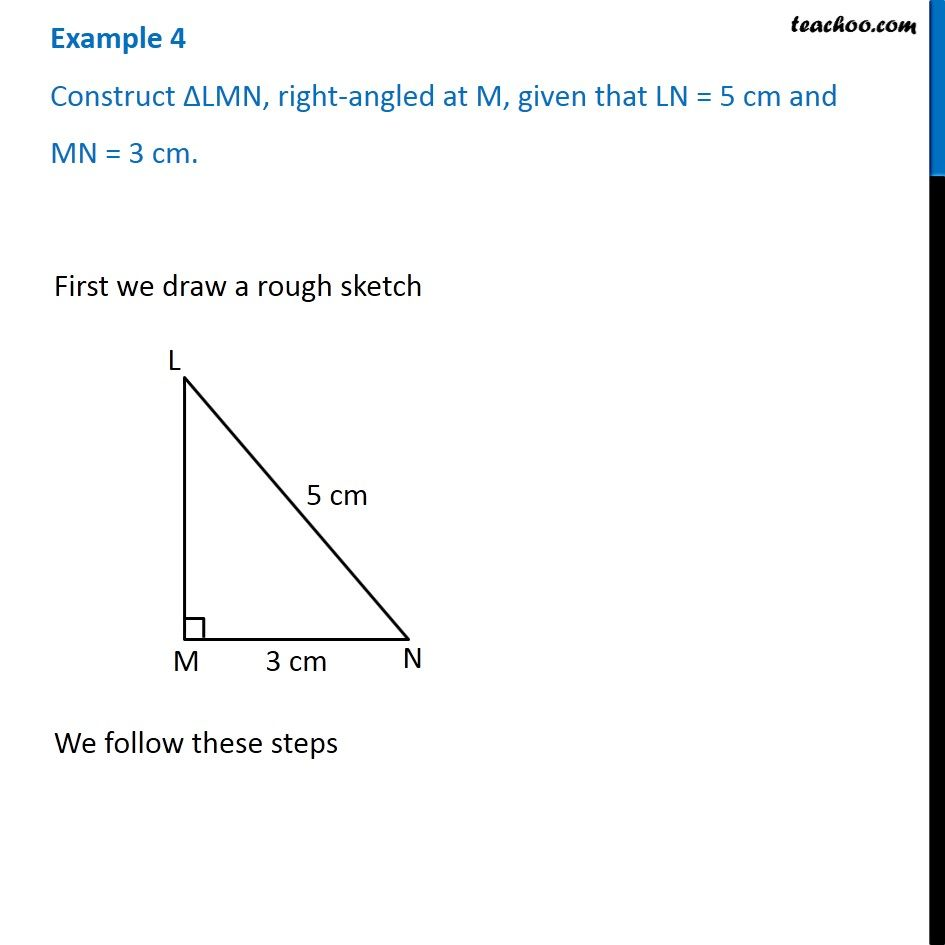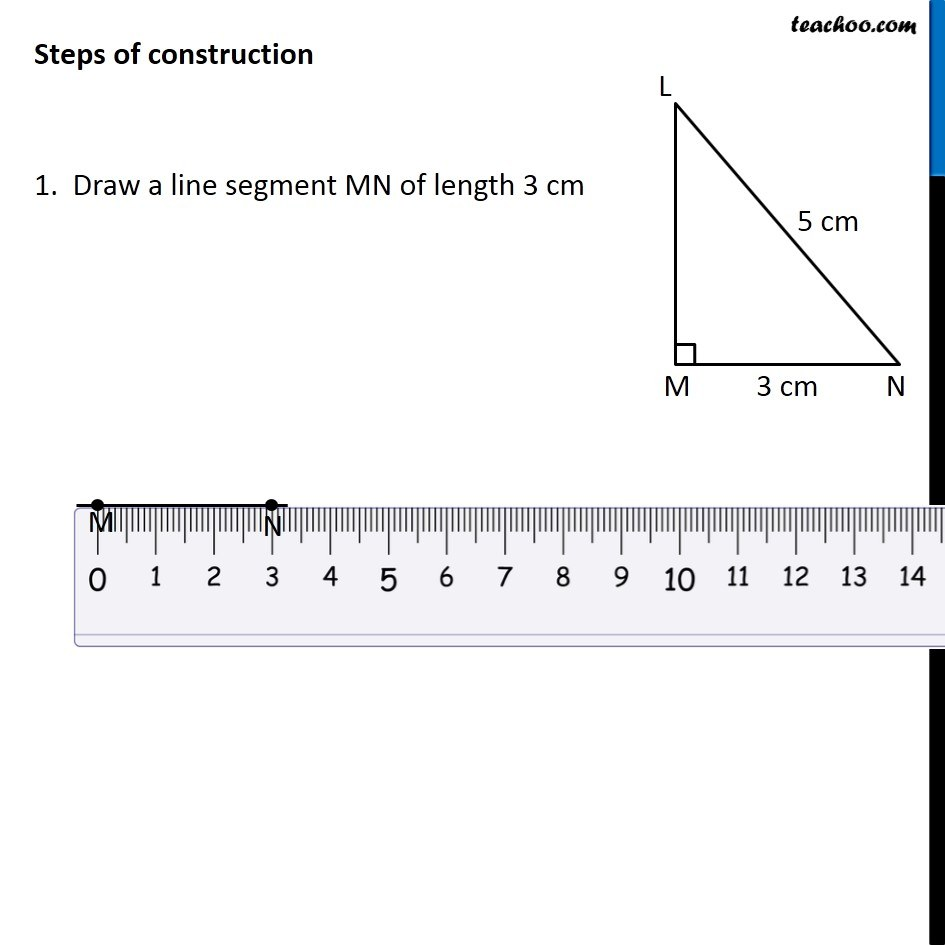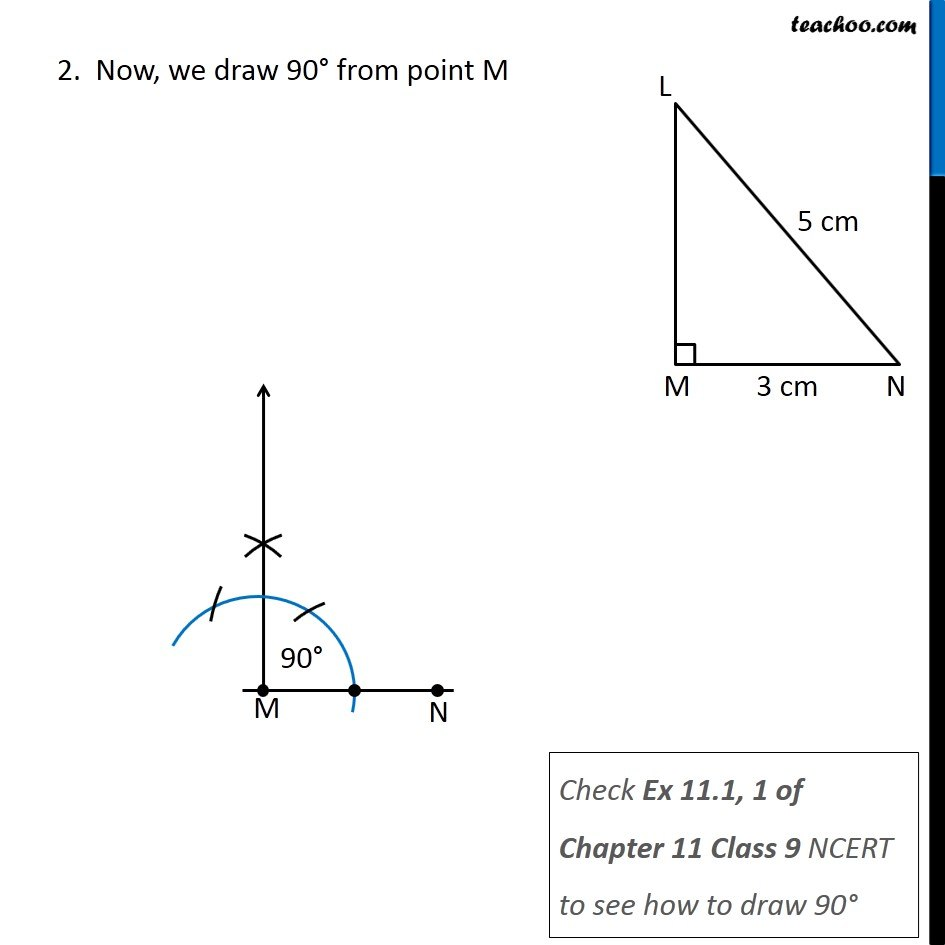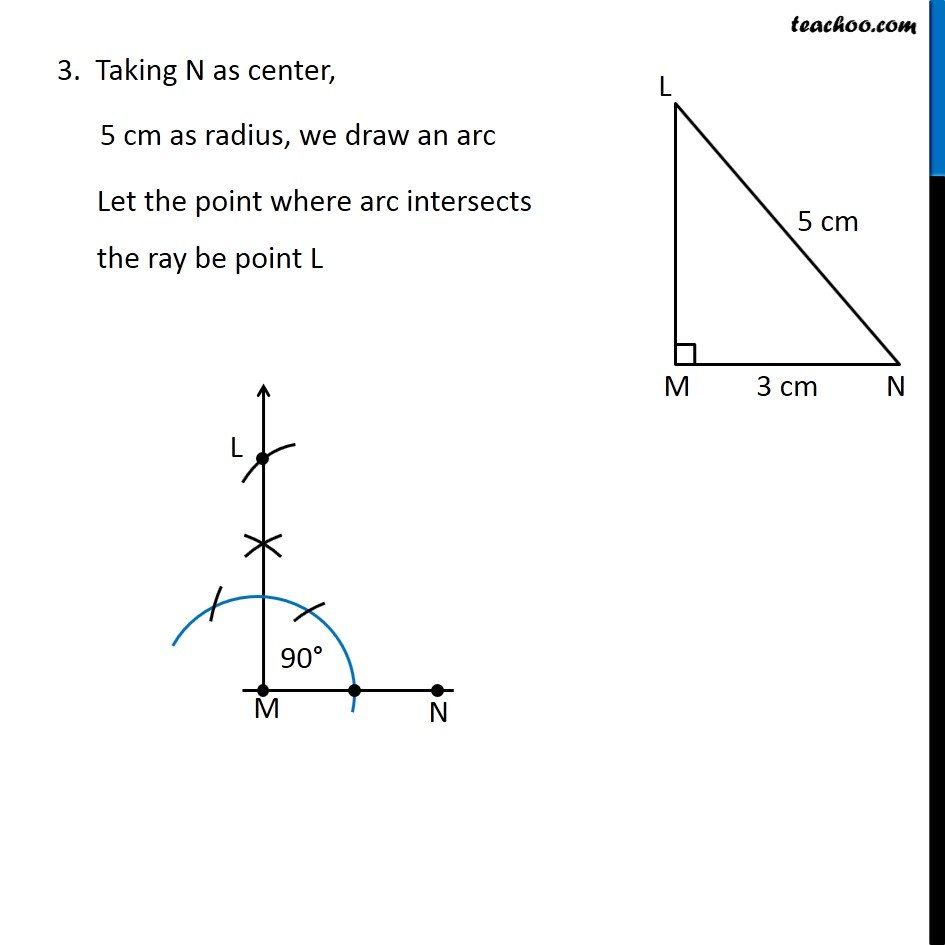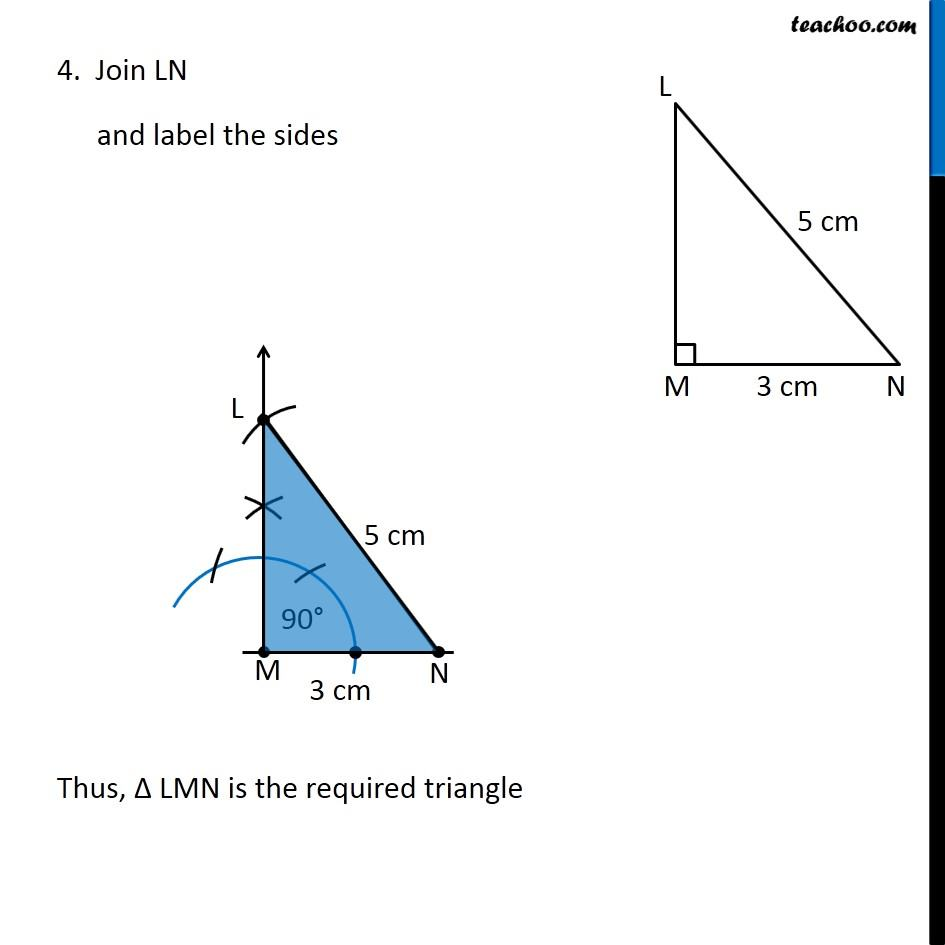Learn in your speed, with individual attention - Teachoo Maths 1-on-1 Class

### Transcript

Example 4 Construct ∆LMN, right-angled at M, given that LN = 5 cm and MN = 3 cm. First we draw a rough sketch We follow these steps Steps of construction 1. Draw a line segment MN of length 3 cm 2. Now, we draw 90° from point M Check Ex 11.1, 1 of Chapter 11 Class 9 NCERT to see how to draw 90° 3. Taking N as center, 5 cm as radius, we draw an arc Let the point where arc intersects the ray be point L 4. Join LN and label the sides Thus, Δ LMN is the required triangle